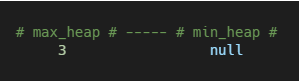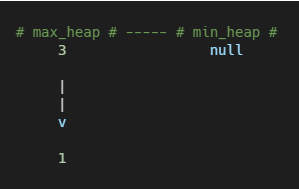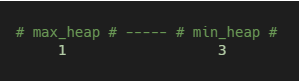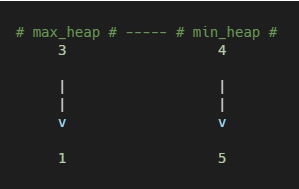# Coding Patterns: Two Heaps

In Coding Patterns series, we will try to recognize common patterns underlying behind each algorithm question, using real examples from Leetcode.

Previous posts were about Sliding Window, Two Pointers, Fast & Slow Pointers, Merge Intervals, Cyclic Sort, In-place Reversal of a Linked List, Breadth First Search (BFS) and Depth First Search (DFS) patterns and today, we will introduce Two Heaps pattern which is very useful to solve the problems where we are given a set of elements such that we can divide them into two parts.

To be able to solve these kinds of problems, we want to know the smallest element in one part and the biggest element in the other part. Two Heaps pattern uses two Heap data structure to solve these problems; a Min Heap to find the smallest element and a Max Heap to find the biggest element.

## Problem: Find Median from Data Stream

LeetCode 295 - Find Median from Data Stream [hard]

Median is the middle value in an ordered integer list. If the size of the list is even, there is no middle value. So the median is the mean of the two middle value.

For example:

`[2, 3, 4]`, the median is 3

`[2, 3]`, the median is `(2 + 3) / 2 = 2.5`

Design a data structure that supports the following two operations:

• `void addNum(int num)` - Add a integer number from the data stream to the data structure.
• `double findMedian()` - Return the median of all elements so far.

Example:

``````addNum(1)
findMedian() -> 1.5
findMedian() -> 2
``````

### Two Heaps Solution

A brute force solution could be maintaining a sorted list of all numbers and returning the median whenever required. Inserting a number in a sorted list will take O(N) time if there are N numbers in the list.

A better approach is using the Two Heaps pattern with a `max_heap` and `min_heap`.

Let’s assume that `x` is the median of the list. This means that, half of the items in the list are smaller than (or equal to) `x` and other half is greater than (or equal to) `x`.

1. We can store the smaller part of the list in a `max_heap`. We are using `max_heap` because we are only interested in knowing the largest number in the first half of the list.
2. We can store the larger part of the list in a `min_heap`. We are using `min_heap` because we are only interested in knowing the smallest number in the second half of the list.
3. Inserting a number in a heap will take O(log N) (better than the brute force approach)
4. The median of the current list of numbers can be calculated from the top element of the two heaps.

For Example;

1- `addNum(3)`: We can insert it to `max_heap`. After every insertion, we will balance the number of elements in both heaps to make the elements in them equal. If the count of elements is odd, let’s decide to have more elements in `max_heap` than the `min_heap`.2- `addNum(1)`: Since 1 is smaller than 3, let’s insert it into `max_heap`.3- At this point we need to balance the heaps. Let’s take the largest element from the `max_heap` and insert it into the `min_heap`.4- `findMedian()`: As we have an even number of elements, the median will be the average of the top elements of the heaps:

`(1 + 3) / 2 = 2.0`

5- `addNum(5)`: As 5 is greater than the top element of the `max_heap`, let’s insert it into the `min_heap`. After the insertion, the total count of elements will be odd. As we had decided (step #1) to have more numbers in the `max_heap` in case of odd count, we can take the top (smallest) number from the `min_heap` and insert it into the `max_heap`.6- `findMedian()`: Since we have an odd number of elements, the median will be the top element of `max_heap` which is 3. Odd number of elements is always mean that, `max_heap` has one extra element than `min_heap`.

7- `insertNum(4)`: Insert 4 into `min_heap`.8- `findMedian()`: There are even number of elements, the median will be the average of the top element of both the heaps.

`(3 + 4) / 2 = 3.5`

``````from heapq import *

class MedianFinder:

def __init__(self):
self.max_heap = []  # containing first half of numbers
self.min_heap = []  # containing second half of numbers

def addNum(self, num: int) -> None:
if not self.max_heap or -self.max_heap >= num:
heappush(self.max_heap, -num)
else:
heappush(self.min_heap, num)

# either both heaps will have equal number of elements or max-heap will have one more element
if len(self.max_heap) > len(self.min_heap) + 1:
heappush(self.min_heap, -heappop(self.max_heap))
elif len(self.max_heap) < len(self.min_heap):
heappush(self.max_heap, -heappop(self.min_heap))

def findMedian(self) -> float:
# we have even number of elements, take the average of middle two elements
if len(self.max_heap) == len(self.min_heap):
return -self.max_heap / 2.0 + self.min_heap / 2.0
# we have odd number of elements, the first element in max-heap is the median element
return -float(self.max_heap)
``````

Time Complexity: O(log N) for `addNum()` and O(1) for `findMedian()`.

Space Complexity: O(N)

## How to identify?

This approach is quite useful when dealing with the problems where we are given a set of elements such that we can divide them into two parts.

To be able to solve these kinds of problems, we want to know the smallest element in one part and the biggest element in the other part. Two Heaps pattern uses two Heap data structure to solve these problems; a Min Heap to find the smallest element and a Max Heap to find the biggest element.

Tags:

Categories:

Updated: